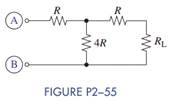Create an Account

Home / Questions / Select the value of R in Figure P2−55 so that R AB = R L .

Select the value of R in Figure P2−55 so that R AB = R L .

Select the value of R in Figure P2−55 so that RAB = RL.Jul 28 2020 View more View LessSubscribe To Get Solution